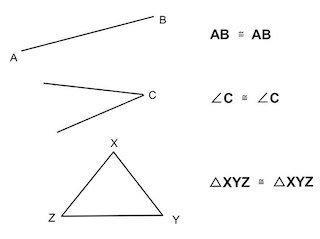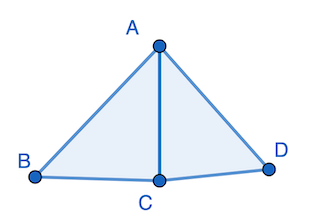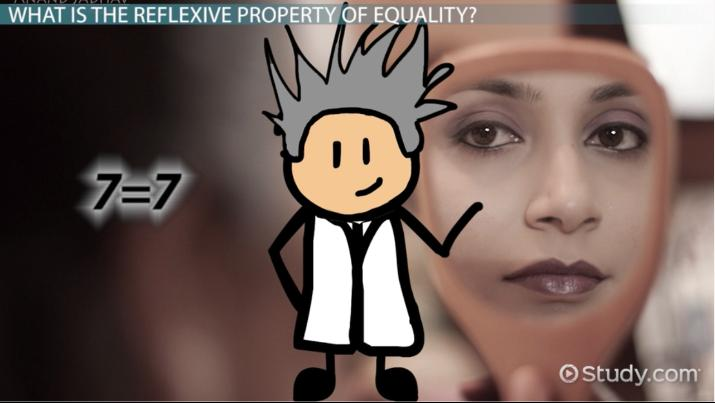# The Reflexive Property of Equality

Stephanie Stanglin, Karin Gonzalez, Laura Pennington
• Author
Stephanie Stanglin

Stephanie Stanglin is a license secondary mathematics teacher with 4.5 years experience as math teacher, .66 years as a K-12 mathematics coach, and .33 years as a 3-10 mathematics tutor. She holds a B.A. in Mathematics Education, a M.S.Ed. in Educational Leadership, and is finishing an M.Ed. in Curriculum and Instruction, STEM concentration.

• Instructor
Karin Gonzalez

Karin has taught middle and high school Health and has a master's degree in social work.

• Expert Contributor
Laura Pennington

Laura received her Master's degree in Pure Mathematics from Michigan State University, and her Bachelor's degree in Mathematics from Grand Valley State University. She has 20 years of experience teaching collegiate mathematics at various institutions.

Learn about the reflexive property of equality. Understand reflexive property’s significance and solve some examples and proofs of the reflexive property. Updated: 01/17/2022

Show

## What is the Reflexive Property of Equality?

The Reflexive Property of Equality means that a quantity is equal to itself and in the same order. It is useful because it provides clarity and consistency in math for people to know that something is equal to itself (4=4, x=x, a+b=a+b).

This property is used mainly in geometry to help in proofs by stating that something is equal to itself to help prove another statement in a geometric proof.Note: The equals sign with the tilde on top means that the two quantities are congruent.

For example, a proof might state that line segment AC is equal to line segment AC, which could be useful in proving the congruence of two connected shapes.If more is known about the other parts of the triangles (congruent angles or sides), the triangles could be proven congruent using the Reflexive Property of Equality with AC.

### Uses For the Reflexive Property

The Reflexive Property of Equality is mainly used to help prove other statements in geometric proofs. It is connected to three other properties of equality: symmetric, transitive, and substitution.

Symmetric Property of Equality: A quantity is equal to its reflection (i.e. 4+3=3+4, a+b+c=c+b+a). The Symmetric Property of Equality is related to the Reflexive Property of Equality because it has to do with quantities that are equal to itself.

Example: 17=17 can be the Reflexive Property of Equality because it is a quantity equal to itself and in the same order, and the Symmetric Property of Equality because 17 reflects 17. They differ when there is more than one term on each side since b+c=b+c can only be the Reflexive Property of Equality because it is in the same order on both sides. In contrast, b+c=c+b can only be the Symmetric Property of Equality because it is in the opposite order on each side.

Transitive Property of Equality: If one quantity is equal to a second quantity and the second quantity is equal to a third quantity, then the first quantity is equal to the third quantity (i.e., if a=b and b=c, then a=c). The Transitive Property of Equality is related to the Reflexive Property of Equality because both have to do with related quantities that are equal to each other.

Example: 98=98 can be the Reflexive Property of Equality because it is a quantity equal to itself and in the same order, and the Transitive Property of Equality because if 98=98 and 98=98, then 98=98. This type of example would never really be used with the Transitive Property of Equality, but it shows that these properties are related. They differ when there are different quantities on each side of the equal sign because that cannot be the Reflexive Property of Equality.An error occurred trying to load this video.

Try refreshing the page, or contact customer support.

Coming up next: X Variable: Definition & Concept

### You're on a roll. Keep up the good work!

Replay
Your next lesson will play in 10 seconds
• 0:01 Reflexive Property of Equality
• 1:01 Importance
• 1:38 Examples
• 2:22 Symmetric Property of Equality
• 2:54 Lesson Summary
Save Save

Want to watch this again later?

Timeline
Autoplay
Autoplay
Speed Speed

To unlock this lesson you must be a Study.com Member.

• Activities
• FAQs

## Using the Reflexive Property to Prove Other Properties of Equality

### Three Properties of Equality

• The reflexive property states that any real number, a, is equal to itself. That is, a = a.
• The symmetric property states that for any real numbers, a and b, if a = b then b = a.
• The transitive property states that for any real numbers, a, b, and c, if a = b and b = c, then a = c.

### Fill-in-the-Blanks Activity

#### Proof of the Symmetric Property:

To prove the symmetric property, we will assume that a and b are real numbers and that a = b, then we will show that b = a. By the _____ property, we know that since a is a real number, it must be the case that a = a. We are given that a = b. Therefore, we can plug b into either side of the equation a = a for a, and still have a true statement. If we plug b into the left-hand side of a = a, we get _____ = a. Thus, we have that if a = b, then it must also be true that b = a. This proves the symmetric property that if a = b, then b = _____.

#### Proof of the Transitive Property:

To prove the transitive property, we will assume that a, b, and c are real numbers and that a = b and b = c, then we will show that a = c. Since we have that a = b, the _____ property gives that b = a. Furthermore, the reflexive property gives that b = _____. Thus, we have that b = a, b = c, and b = b. Since b = a, we can plug a into the equation b = b for b on the left-hand side of the equation, and since b = c, we can plug c into the equation for b on the right-hand side of the equation. This gives _____ = _____. Thus, we have that if a = b and b = c, then a = c, proving the transitive property.

Proof of the Symmetric Property

• Blank 1: Reflexive
• Blank 2: b
• Blank 3: a

Proof of the Transitive Property

• Blank 1: Symmetric
• Blank 2: b
• Blank 3: a
• Blank 4: c

#### What is reflexive property of equation?

The reflexive property of equation is when one side of the equal sign is the exact same as the other side. The two sides need to be of the same value and in the same order in order them to be reflexive.

#### What is reflexive property in geometry?

The reflexive property in geometry is when a quantity is equal to itself (and in the same order). It is used to prove a statement in a larger geometric proof, usually dealing with congruent shapes.

#### What is the reflexive property of equality?

The reflexive property of equality is a property that shows that something is equal or congruent to itself. It provides the basis that values that are the same are equivalent, which is helpful in many mathematical concepts.

#### What are some examples of reflexive property?

More simple examples could be 76=76 and d=d because there is only one term on each side and they are the same term, which means they have the same value. More complex examples could be x+4=x+4 and 39-a+b=39-a+b because the same terms are on each side (so the two sides have the same value) and they are in the same order.

### Register to view this lesson

Are you a student or a teacher?